# 八、检测和跟踪不同的身体部位

• 如何使用 Haar 级联
• 什么是完整图片
• 什么是自适应提升
• 如何在实时视频流中检测和跟踪人脸
• 如何在实时视频流中检测和跟踪眼睛
• 如何将太阳镜自动覆盖在人的脸上
• 如何检测耳朵，鼻子和嘴巴
• 如何使用形状分析检测瞳孔

# 什么是完整图像？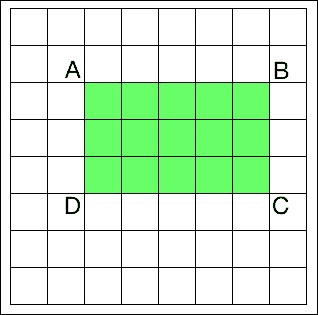矩形 ABCD 的面积 = AC – (AB + AD - AA)


# 检测和跟踪人脸

OpenCV 提供了一个不错的检测框架。 我们只需要加载级联文件，然后就可以使用它来检测图像中的人脸。 让我们看看如何做到这一点：

import cv2
import numpy as np

cap = cv2.VideoCapture(0)
scaling_factor = 0.5

while True:
frame = cv2.resize(frame, None, fx=scaling_factor, fy=scaling_factor, interpolation=cv2.INTER_AREA)
gray = cv2.cvtColor(frame, cv2.COLOR_BGR2GRAY)

for (x,y,w,h) in face_rects:
cv2.rectangle(frame, (x,y), (x+w,y+h), (0,255,0), 3)

cv2.imshow('Face Detector', frame)

c = cv2.waitKey(1)
if c == 27:
break

cap.release()
cv2.destroyAllWindows()# 人脸上的乐趣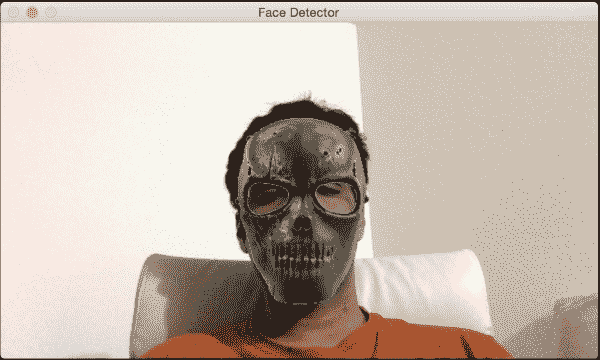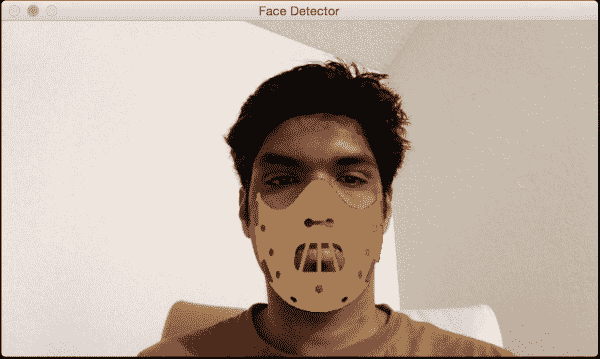import cv2
import numpy as np

cap = cv2.VideoCapture(0)
scaling_factor = 0.5

while True:
frame = cv2.resize(frame, None, fx=scaling_factor, fy=scaling_factor, interpolation=cv2.INTER_AREA)
gray = cv2.cvtColor(frame, cv2.COLOR_BGR2GRAY)

for (x,y,w,h) in face_rects:
if h > 0 and w > 0:
# Adjust the height and weight parameters depending on the sizes and the locations. You need to play around with these to make sure you get it right.
h, w = int(1.4*h), int(1.0*w)
y -= 0.1*h

# Extract the region of interest from the image
frame_roi = frame[y:y+h, x:x+w]

# Convert color image to grayscale and threshold it

# Use the mask to extract the face mask region of interest

# Use the inverse mask to get the remaining part of the image

# add the two images to get the final output

cv2.imshow('Face Detector', frame)

c = cv2.waitKey(1)
if c == 27:
break

cap.release()
cv2.destroyAllWindows()


## 底层原理

frame_roi = frame[y:y+h, x:x+w]


# 检测眼睛

import cv2
import numpy as np

cap = cv2.VideoCapture(0)
ds_factor = 0.5

while True:
frame = cv2.resize(frame, None, fx=ds_factor, fy=ds_factor, interpolation=cv2.INTER_AREA)
gray = cv2.cvtColor(frame, cv2.COLOR_BGR2GRAY)

for (x,y,w,h) in faces:
roi_gray = gray[y:y+h, x:x+w]
roi_color = frame[y:y+h, x:x+w]
for (x_eye,y_eye,w_eye,h_eye) in eyes:
center = (int(x_eye + 0.5*w_eye), int(y_eye + 0.5*h_eye))
radius = int(0.3 * (w_eye + h_eye))
color = (0, 255, 0)
thickness = 3

cv2.imshow('Eye Detector', frame)

c = cv2.waitKey(1)
if c == 27:
break

cap.release()
cv2.destroyAllWindows()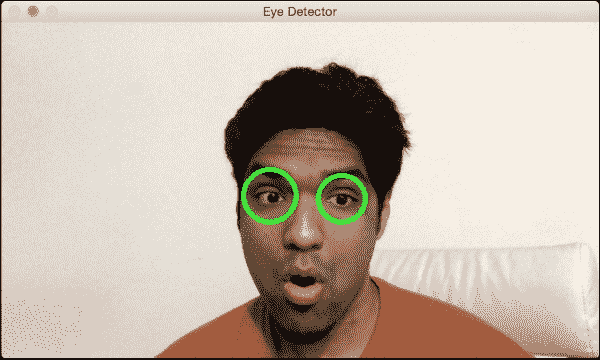# 有趣的眼睛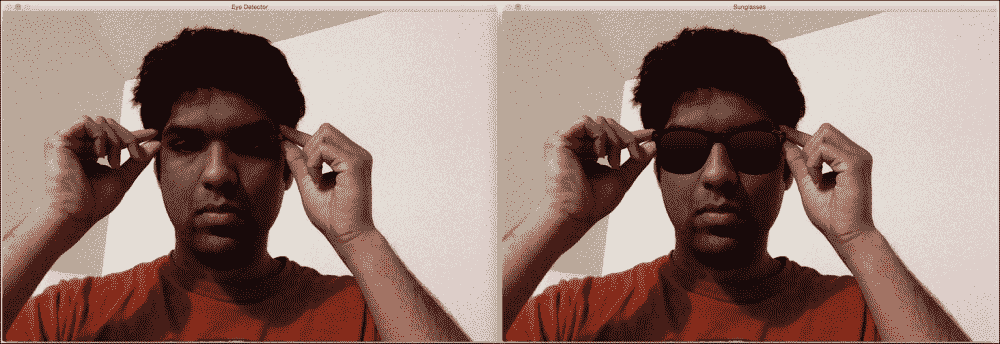import cv2
import numpy as np

gray = cv2.cvtColor(img, cv2.COLOR_BGR2GRAY)

centers = []

for (x,y,w,h) in faces:
roi_gray = gray[y:y+h, x:x+w]
roi_color = img[y:y+h, x:x+w]
for (x_eye,y_eye,w_eye,h_eye) in eyes:
centers.append((x + int(x_eye + 0.5*w_eye), y + int(y_eye + 0.5*h_eye)))

if len(centers) > 0:
# Overlay sunglasses; the factor 2.12 is customizable depending on the size of the face
sunglasses_width = 2.12 * abs(centers - centers)
overlay_img = np.ones(img.shape, np.uint8) * 255
h, w = sunglasses_img.shape[:2]
scaling_factor = sunglasses_width / w
overlay_sunglasses = cv2.resize(sunglasses_img, None, fx=scaling_factor,
fy=scaling_factor, interpolation=cv2.INTER_AREA)

x = centers if centers < centers else centers

# customizable X and Y locations; depends on the size of the face
x -= 0.26*overlay_sunglasses.shape
y += 0.85*overlay_sunglasses.shape

h, w = overlay_sunglasses.shape[:2]
overlay_img[y:y+h, x:x+w] = overlay_sunglasses

gray_sunglasses = cv2.cvtColor(overlay_img, cv2.COLOR_BGR2GRAY)
ret, mask = cv2.threshold(gray_sunglasses, 110, 255, cv2.THRESH_BINARY)

cv2.imshow('Eye Detector', img)
cv2.imshow('Sunglasses', final_img)
cv2.waitKey()
cv2.destroyAllWindows()


# 检测耳朵

import cv2
import numpy as np

gray = cv2.cvtColor(img, cv2.COLOR_BGR2GRAY)

for (x,y,w,h) in left_ear:
cv2.rectangle(img, (x,y), (x+w,y+h), (0,255,0), 3)

for (x,y,w,h) in right_ear:
cv2.rectangle(img, (x,y), (x+w,y+h), (255,0,0), 3)

cv2.imshow('Ear Detector', img)
cv2.waitKey()
cv2.destroyAllWindows()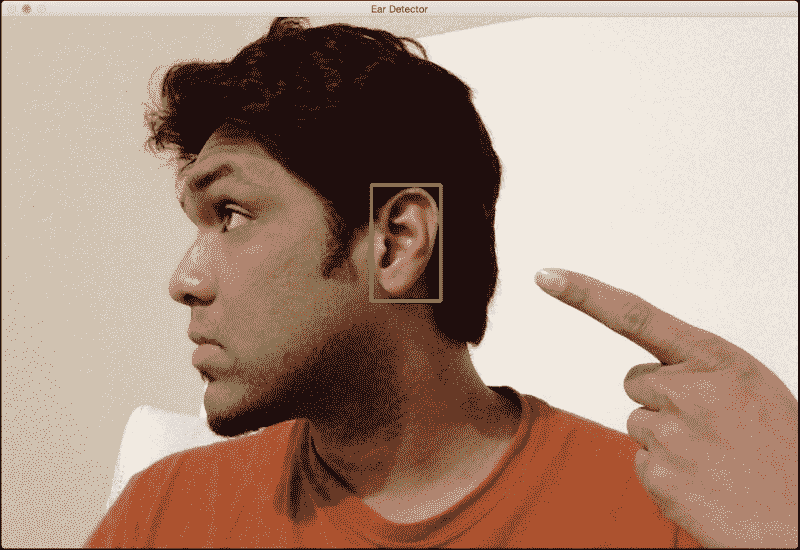# 检测嘴巴

import cv2
import numpy as np

cap = cv2.VideoCapture(0)
ds_factor = 0.5

while True:
frame = cv2.resize(frame, None, fx=ds_factor, fy=ds_factor, interpolation=cv2.INTER_AREA)
gray = cv2.cvtColor(frame, cv2.COLOR_BGR2GRAY)

for (x,y,w,h) in mouth_rects:
y = int(y - 0.15*h)
cv2.rectangle(frame, (x,y), (x+w,y+h), (0,255,0), 3)
break

cv2.imshow('Mouth Detector', frame)

c = cv2.waitKey(1)
if c == 27:
break

cap.release()
cv2.destroyAllWindows()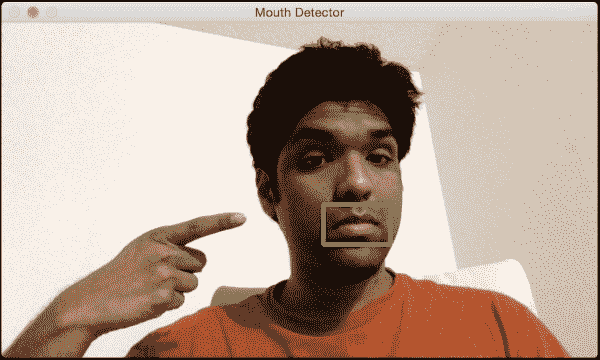# 现在是覆盖胡子的时候了

import cv2
import numpy as np

cap = cv2.VideoCapture(0)
scaling_factor = 0.5

while True:
frame = cv2.resize(frame, None, fx=scaling_factor, fy=scaling_factor, interpolation=cv2.INTER_AREA)
gray = cv2.cvtColor(frame, cv2.COLOR_BGR2GRAY)

if len(mouth_rects) > 0:
(x,y,w,h) = mouth_rects
h, w = int(0.6*h), int(1.2*w)
x -= 0.05*w
y -= 0.55*h
frame_roi = frame[y:y+h, x:x+w]

cv2.imshow('Moustache', frame)

c = cv2.waitKey(1)
if c == 27:
break

cap.release()
cv2.destroyAllWindows()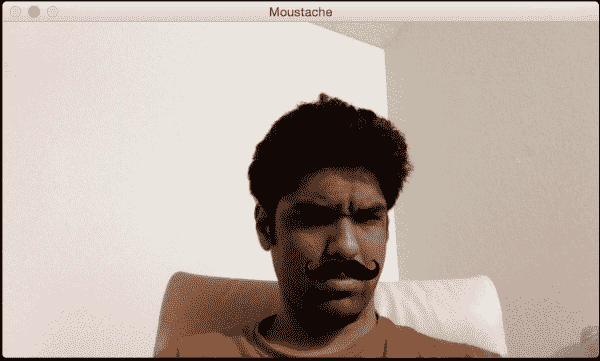# 检测鼻子

import cv2
import numpy as np

cap = cv2.VideoCapture(0)
ds_factor = 0.5

while True:
frame = cv2.resize(frame, None, fx=ds_factor, fy=ds_factor, interpolation=cv2.INTER_AREA)
gray = cv2.cvtColor(frame, cv2.COLOR_BGR2GRAY)

for (x,y,w,h) in nose_rects:
cv2.rectangle(frame, (x,y), (x+w,y+h), (0,255,0), 3)
break

cv2.imshow('Nose Detector', frame)

c = cv2.waitKey(1)
if c == 27:
break

cap.release()
cv2.destroyAllWindows()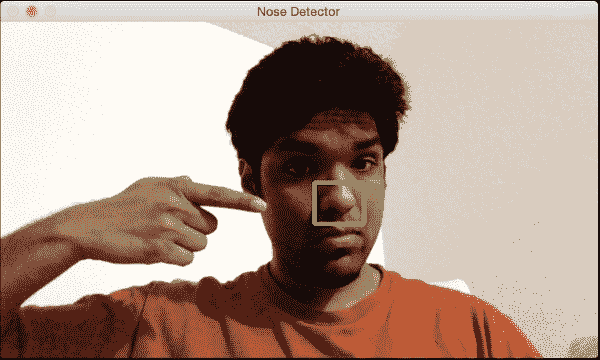# 检测学生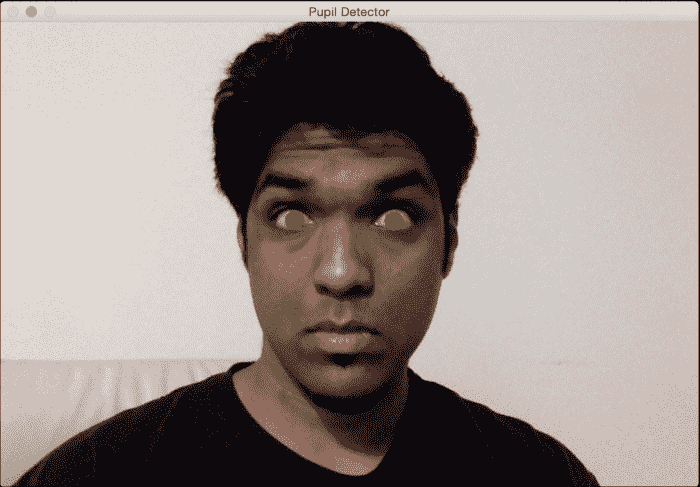import math

import cv2
import numpy as np

scaling_factor = 0.7

img = cv2.resize(img, None, fx=scaling_factor, fy=scaling_factor, interpolation=cv2.INTER_AREA)
cv2.imshow('Input', img)
gray = cv2.cvtColor(~img, cv2.COLOR_BGR2GRAY)

ret, thresh_gray = cv2.threshold(gray, 220, 255, cv2.THRESH_BINARY)
contours, hierarchy = cv2.findContours(thresh_gray, cv2.RETR_EXTERNAL, cv2.CHAIN_APPROX_NONE)

for contour in contours:
area = cv2.contourArea(contour)
rect = cv2.boundingRect(contour)
x, y, width, height = rect
radius = 0.25 * (width + height)

area_condition = (100 <= area <= 200)
symmetry_condition = (abs(1 - float(width)/float(height)) <= 0.2)
fill_condition = (abs(1 - (area / (math.pi * math.pow(radius, 2.0)))) <= 0.3)

if area_condition and symmetry_condition and fill_condition:

cv2.imshow('Pupil Detector', img)

c = cv2.waitKey()
cv2.destroyAllWindows()


## 解构代码

gray = cv2.cvtColor(~img, cv2.COLOR_BGR2GRAY)